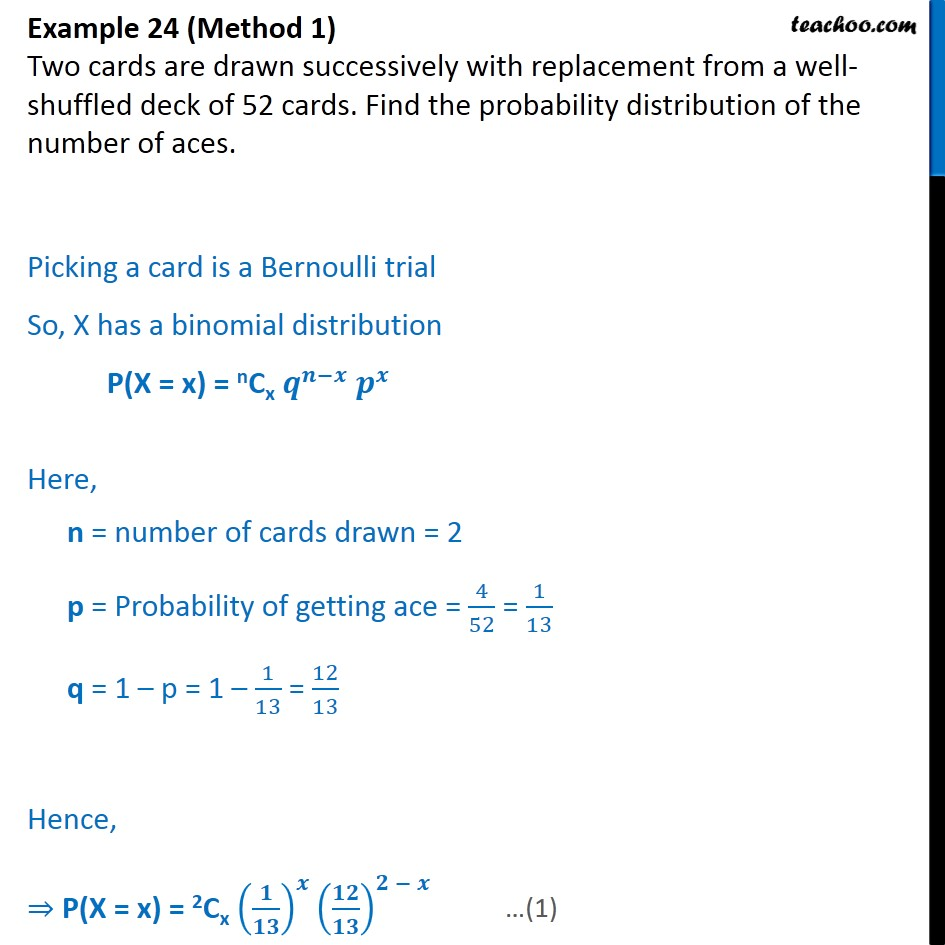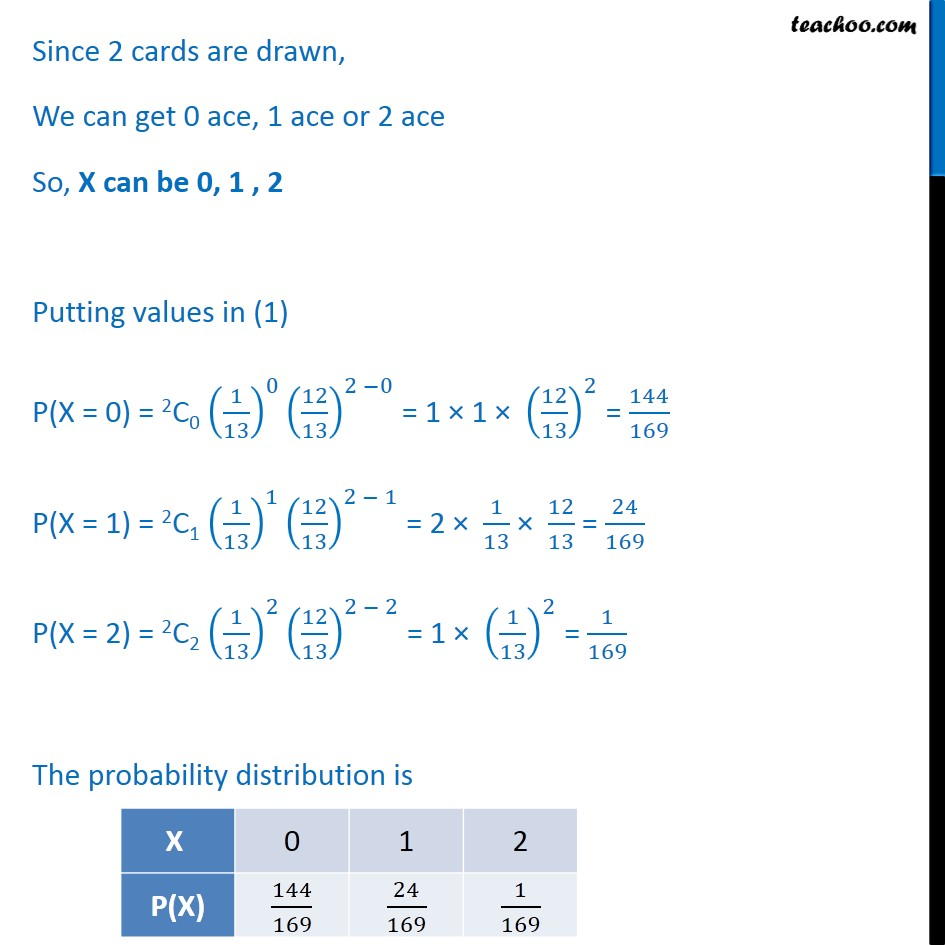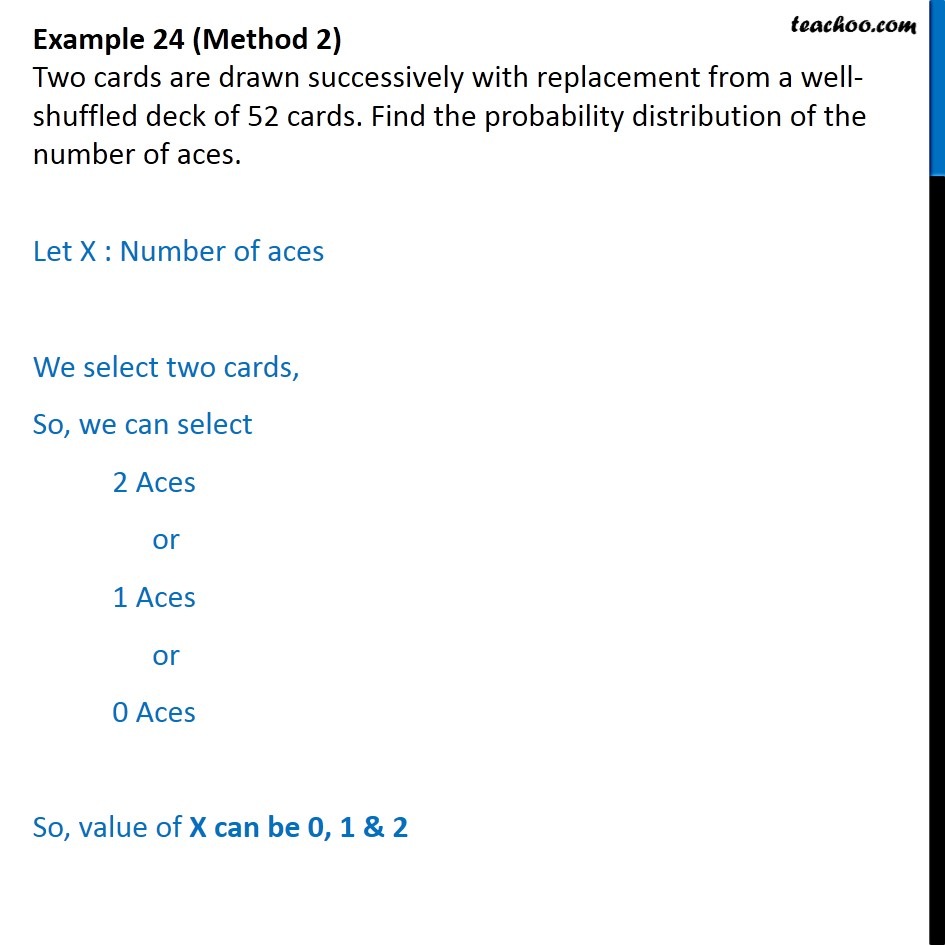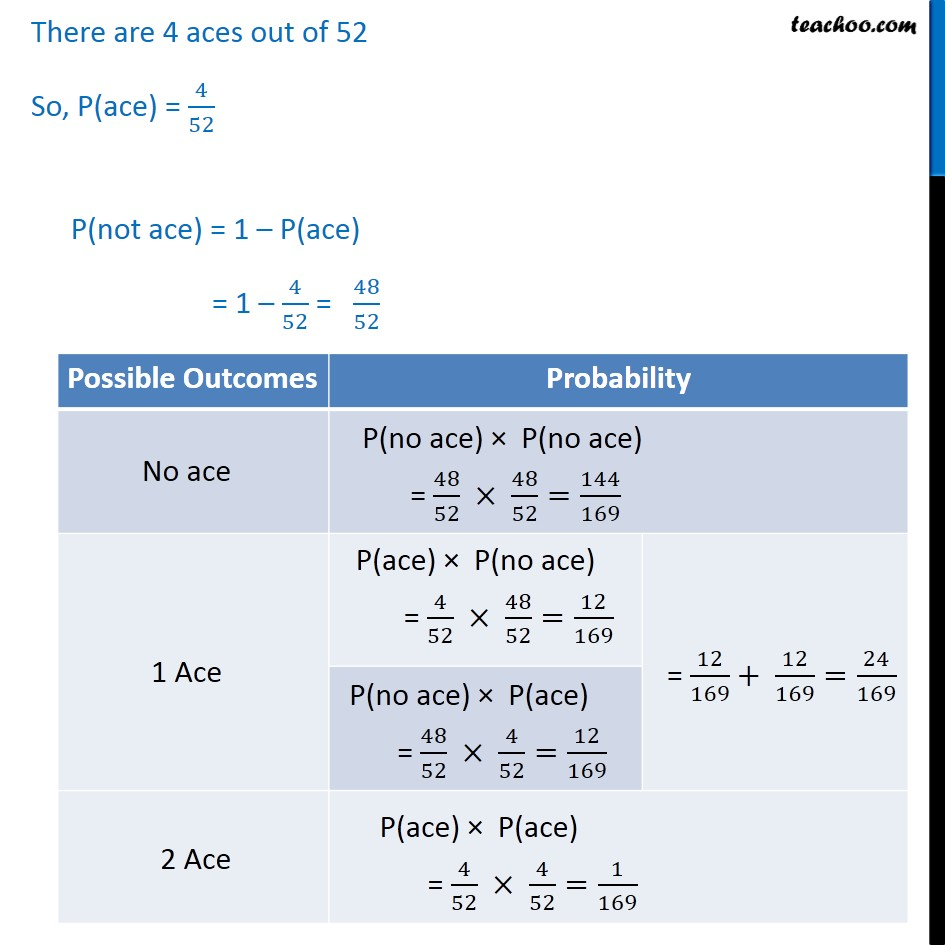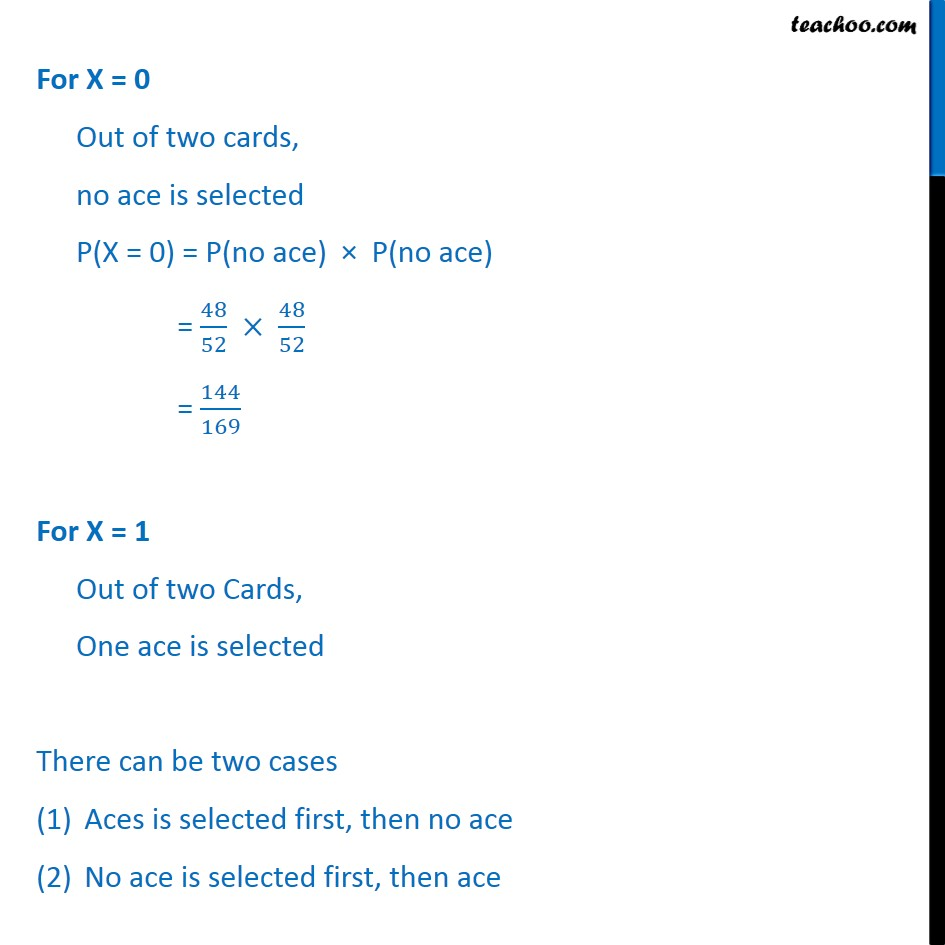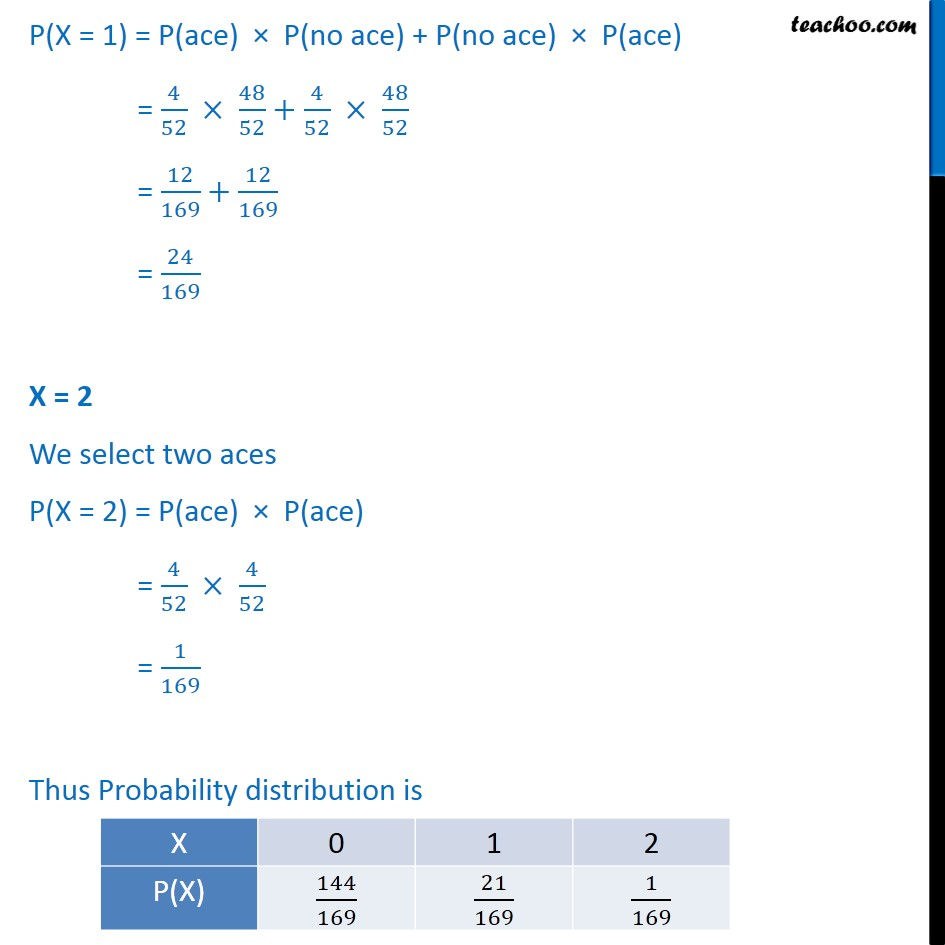1. Chapter 13 Class 12 Probability
2. Serial order wise
3. Examples

Transcript

Example 24 (Method 1) Two cards are drawn successively with replacement from a well-shuffled deck of 52 cards. Find the probability distribution of the number of aces. Picking a card is a Bernoulli trial So, X has a binomial distribution P(X = x) = nCx Here, n = number of cards drawn = 2 p = Probability of getting ace = 4 52 = 1 13 q = 1 p = 1 1 13 = 12 13 Hence, P(X = x) = 2Cx Since 2 cards are drawn, We can get 0 ace, 1 ace or 2 ace So, X can be 0, 1 , 2 Putting values in (1) P(X = 0) = 2C0 1 13 0 12 13 2 0 = 1 1 12 13 2 = 144 169 P(X = 1) = 2C1 1 13 1 12 13 2 1 = 2 1 13 12 13 = 24 169 P(X = 2) = 2C2 1 13 2 12 13 2 2 = 1 1 13 2 = 1 169 The probability distribution is Example 24 (Method 2) Two cards are drawn successively with replacement from a well-shuffled deck of 52 cards. Find the probability distribution of the number of aces. Let X : Number of aces We select two cards, So, we can select 2 Aces or 1 Aces or 0 Aces So, value of X can be 0, 1 & 2 There are 4 aces out of 52 So, P(ace) = 4 52 P(not ace) = 1 P(ace) = 1 4 52 = 48 52 For X = 0 Out of two cards, no ace is selected P(X = 0) = P(no ace) P(no ace) = 48 52 48 52 = 144 169 For X = 1 Out of two Cards, One ace is selected There can be two cases Aces is selected first, then no ace No ace is selected first, then ace P(X = 1) = P(ace) P(no ace) + P(no ace) P(ace) = 4 52 48 52 + 4 52 48 52 = 12 169 + 12 169 = 24 169 X = 2 We select two aces P(X = 2) = P(ace) P(ace) = 4 52 4 52 = 1 169 Thus Probability distribution is

Examples